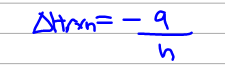# Problem: During an experiment, a student adds 1.23 g of CaO to 200.0 mL of 0.500 M HCI. The student observes a temperature increase of 5.10°C. Assuming the solution's final volume is 200.0 mL, the density is 1.00 g/mL, and the heat capacity is 4.184 J/(g.°C), calculate the heat of the reaction (kJ/mol), ΔHrxn.CaO (s) + 2H+ (aq) → Ca2+ (aq) + H2O (l)

###### FREE Expert Solution
86% (133 ratings)
###### FREE Expert Solution

We can calculate the enthalpy of reaction using the equation below :Here q is the heat absorbed by the solution and n is the number of moles reacted.

Now we have to find out first the limiting reactant in this reaction.

86% (133 ratings)###### Problem Details

During an experiment, a student adds 1.23 g of CaO to 200.0 mL of 0.500 M HCI. The student observes a temperature increase of 5.10°C.

Assuming the solution's final volume is 200.0 mL, the density is 1.00 g/mL, and the heat capacity is 4.184 J/(g.°C), calculate the heat of the reaction (kJ/mol), ΔHrxn.

CaO (s) + 2H+ (aq) → Ca2+ (aq) + H2O (l)

What scientific concept do you need to know in order to solve this problem?

Our tutors have indicated that to solve this problem you will need to apply the Calorimetry concept. You can view video lessons to learn Calorimetry. Or if you need more Calorimetry practice, you can also practice Calorimetry practice problems.

What is the difficulty of this problem?

Our tutors rated the difficulty ofDuring an experiment, a student adds 1.23 g of CaO to 200.0 ...as high difficulty.

How long does this problem take to solve?

Our expert Chemistry tutor, Dasha took 12 minutes and 59 seconds to solve this problem. You can follow their steps in the video explanation above.

What professor is this problem relevant for?

Based on our data, we think this problem is relevant for Professor Arasasingham's class at UCI.